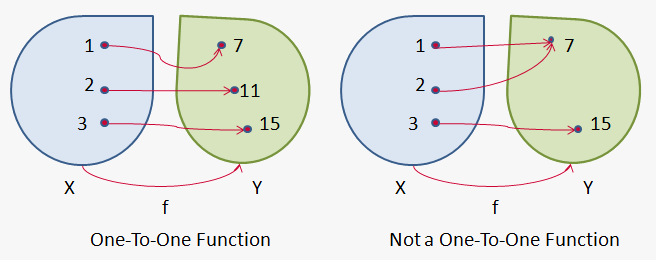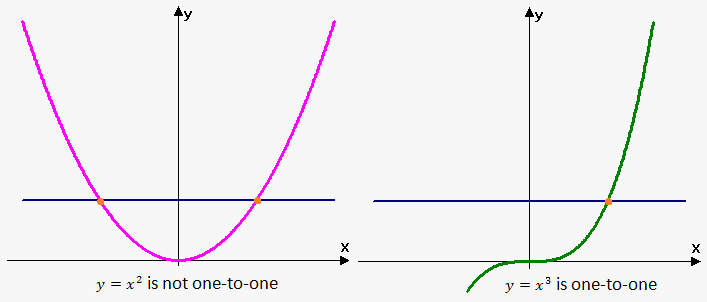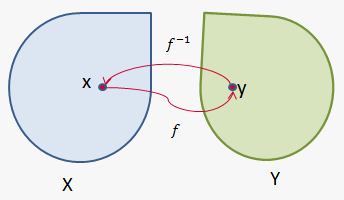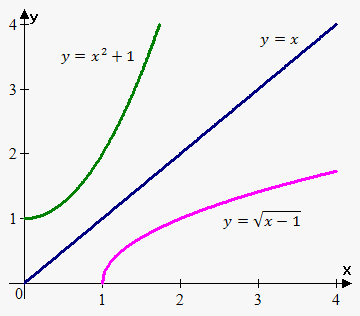# Inverse of a Function

We already know that a function is a rule that allows finding for every value of ${x}$ the corresponding value of ${y}$. But what if we are given a rule, i.e. a function, and the value of ${y}$ and we want to find the corresponding value of ${x}$?

In other words, we want to find a function that will express ${x}$ in terms of ${y}$. Such a function is called an inverse function and is denoted by ${{f}}^{{-{1}}}$.

If ${y}={f{{\left({x}\right)}}}$, the inverse function is ${x}={{f}}^{{-{1}}}{\left({y}\right)}$.

For example, if ${y}={f{{\left({x}\right)}}}={{x}}^{{3}}$, it can be stated that ${{f}}^{{-{1}}}{\left({27}\right)}={3}$, because ${f{{\left({3}\right)}}}={27}$.

Not all functions have a unique inverse. Let's take the function ${f{{\left({x}\right)}}}={{x}}^{{2}}$. Since ${f{{\left(-{2}\right)}}}={4}$ and ${f{{\left({2}\right)}}}={4}$, it can be concluded that ${{f}}^{{-{1}}}{\left({4}\right)}$ is undefined, because there are 2 values that correspond to ${4}$, namely ${2}$ and $-{2}$. Therefore, the inverse of ${y}={{x}}^{{2}}$ is a multi-valued function. In this case, we will say that the function doesn't have an inverse, because the inverse is a multi-valued function, which we don't consider to be a function.

So, a function will have an inverse only when for different values of ${x}$ we have different values of ${y}$.

Definition: a function ${f{}}$ is called one-to-one (or injective), if it never takes the same value twice, i.e. ${f{{\left({x}_{{1}}\right)}}}\ne{f{{\left({x}_{{2}}\right)}}}$ whenever ${x}_{{1}}\ne{x}_{{2}}$.One-to-one functions are precisely the functions that possess inverse functions.

From this definition, it follows that ${y}={{x}}^{{2}}$ is not a one-to-one function, because ${y}{\left({2}\right)}={y}{\left(-{2}\right)}={4}$; so, different values have the same output.

On the other hand, ${y}={{x}}^{{3}}$ is a one-to-one function, because ${{x}_{{1}}^{{3}}}\ne{{x}_{{2}}^{{3}}}$ whenever ${x}_{{1}}\ne{x}_{{2}}$ (different values can't have the same cube). Thus, ${y}={{x}}^{{3}}$ has an inverse.

Graphically, we can determine whether a function is one-to-one using the horizontal line test.

Horizontal line test. A function is one-to-one if and only if no horizontal line intersects its graph more than once.Now, let's give a more formal definition of the inverse function.

Definition. Let ${f{}}$ be a one-to-one function with the domain ${X}$ and the range ${Y}$. Then, its inverse function ${{f}}^{{-{1}}}$ has the domain ${Y}$ and the range ${X}$ and is defined as follows: ${{f}}^{{-{1}}}{\left({y}\right)}={x}\ \Leftrightarrow\ {f{{\left({x}\right)}}}={y}$.

Note that the domain of ${f{}}$ equals the range of ${{f}}^{{-{1}}}$ and the range of ${f{}}$ equals the domain of ${{f}}^{{-{1}}}$.

When we want to concentrate on the inverse function, we will usually write ${y}={{f}}^{{-{1}}}{\left({x}\right)}$, i.e. we will interchange ${x}$ and ${y}$, because we traditionally use ${x}$ as an independent variable.

The inverse function means that if we apply ${f{}}$ to ${x}$, we will obtain ${y}$.If we now apply ${{f}}^{{-{1}}}$ to ${y}$, we will arrive back at ${x}$. And vice versa: applying ${{f}}^{{-{1}}}$ to ${y}$ and then applying ${f{}}$ to the result will bring us back to ${y}$.

As a result, we can say that a function and its inverse cancel the influence of each other.

Thus, there are two properties of inverse functions:

1. ${{f}}^{{-{1}}}{\left({f{{\left({x}\right)}}}\right)}={x}$ for any ${x}$ from ${X}$.
2. ${f{{\left({{f}}^{{-{1}}}{\left({x}\right)}\right)}}}={x}$ for any ${x}$ from ${Y}$.

Example 1. Given ${f{{\left({1}\right)}}}={4}$, ${f{{\left({2}\right)}}}={3}$, ${f{{\left({5}\right)}}}={10}$, find ${{f}}^{{-{1}}}{\left({3}\right)}$, ${{f}}^{{-{1}}}{\left({4}\right)}$, ${{f}}^{{-{1}}}{\left({5}\right)}$.

${{f}}^{{-{1}}}{\left({3}\right)}={2}$, because ${f{{\left({2}\right)}}}={3}$.

${{f}}^{{-{1}}}{\left({4}\right)}={1}$, because ${f{{\left({1}\right)}}}={4}$.

${{f}}^{{-{1}}}{\left({5}\right)}$ is undefined, because we are not given the value of ${x}$ for which ${f{{\left({x}\right)}}}={5}$.

Now, let’s see how to compute inverse functions. Since we have ${y}={f{{\left({x}\right)}}}$, we need to express ${x}$ in terms of ${y}$ to obtain ${{f}}^{{-{1}}}$.

Steps for finding an inverse:

1. Write ${y}={f{{\left({x}\right)}}}$.
2. Express ${x}$ in terms of ${y}$ (if possible). You will get ${x}={{f}}^{{-{1}}}{\left({y}\right)}$.
3. To obtain ${y}={{f}}^{{-{1}}}{\left({x}\right)}$, interchange ${x}$ and ${y}$.

Actually, we can first perform step 3 and then step 2, i.e. interchange ${x}$ and ${y}$, and then express ${y}$ in terms of ${x}$.

Example 2. Find the inverse of ${y}={{x}}^{{3}}-{3}$.

1. ${y}={{x}}^{{3}}-{3}$.
2. ${x}={\sqrt[{{3}}]{{{y}+{3}}}}$.
3. ${y}={\sqrt[{{3}}]{{{x}+{3}}}}$ is the inverse.

Let's work another short example.

Example 3. Find the inverse of ${y}=\frac{{{x}+{3}}}{{{2}{x}-{5}}}$.

1. ${y}=\frac{{{x}+{3}}}{{{2}{x}-{5}}}$.
2. ${2}{x}{y}-{5}{y}={x}+{3}$, or ${x}=\frac{{{5}{y}+{3}}}{{{2}{y}-{1}}}$.
3. ${y}=\frac{{{5}{x}+{3}}}{{{2}{x}-{1}}}$ is the inverse.

To verify whether we found the inverse correctly, check if ${f{{\left({{f}}^{{-{1}}}{\left({x}\right)}\right)}}}={x}$ holds.

Here, ${f{{\left({x}\right)}}}=\frac{{{x}+{3}}}{{{2}{x}-{5}}}$, and ${{f}}^{{-{1}}}{\left({x}\right)}=\frac{{{5}{x}+{3}}}{{{2}{x}-{1}}}$; so, ${f{{\left({{f}}^{{-{1}}}{\left({x}\right)}\right)}}}=\frac{{\frac{{{5}{x}+{3}}}{{{2}{x}-{1}}}+{3}}}{{{2}\frac{{{5}{x}+{3}}}{{{2}{x}-{1}}}-{5}}}={x}$.

Thus, we found the inverse correctly.

Interchanging the roles of ${x}$ and ${y}$ gives the opportunity to plot the graph of the inverse function based on the graph of the function ${y}={f{{\left({x}\right)}}}$. Since the point ${\left({x}_{{0}},{y}_{{0}}\right)}$ lies on the graph of ${f{}}$, we have that ${\left({y}_{{0}},{x}_{{0}}\right)}$ lies on the graph of ${{f}}^{{-{1}}}$. So, the graph of the inverse is obtained by reflecting the graph of the function about the line ${y}={x}$.

Example 4. Sketch the graph of ${y}=\sqrt{{{x}-{1}}}$ and its inverse.

The domain of ${y}=\sqrt{{{x}-{1}}}$ is ${x}-{1}\ge{0}$, or ${x}\ge{1}$. The range is ${y}\ge{0}$.This means that the domain of the inverse is ${x}\ge{0}$ and the range is ${y}\ge{1}$.

To find the equation of the inverse, we write ${x}$ in terms of ${y}$: ${{y}}^{{2}}={x}-{1}$, or ${x}={{y}}^{{2}}+{1}$. Now, interchange ${y}$ and ${x}$: ${y}={{x}}^{{2}}+{1}$.

So, the inverse is ${y}={{x}}^{{2}}+{1}$.

Now, we draw the graph of the function ${y}=\sqrt{{{x}-{1}}}$ on the interval ${\left[{1},\infty\right)}$ and then reflect it about the line ${y}={x}$.